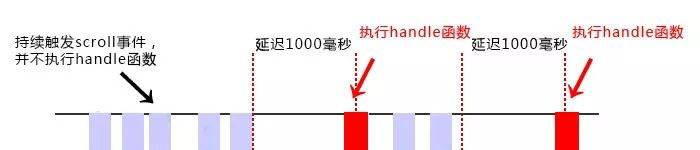# js防抖和节流// 防抖
function debounce(func, wait=0) {    
if (typeof func !== 'function') {
throw new TypeError('need a function arguments')
}
let timeid = null;
let result;

return function() {
let context = this;
let args = arguments;

if (timeid) {
clearTimeout(timeid);
}
timeid = setTimeout(function() {
result = func.apply(context, args);
}, wait);

return result;
}
}
// 处理函数
function handle() {
console.log(Math.random());
}
// 滚动事件
window.addEventListener('scroll', debounce(handle, 1000));var throttle = function(func, delay) {            　　var prev = Date.now();            　　return function() {                　　　　var context = this;                　　　　var args = arguments;                　　　　var now = Date.now();                　　　　if (now - prev >= delay) {                    　　　　　　func.apply(context, args);                    　　　　　　prev = Date.now();                　　　　}            　　}        }        function handle() {            　　console.log(Math.random());        }        window.addEventListener('scroll', throttle(handle, 1000));

// 节流throttle代码（定时器）：
var throttle = function(func, delay) {
var timer = null;
return function() {
var context = this;
var args = arguments;
if (!timer) {
timer = setTimeout(function() {
func.apply(context, args);
timer = null;
}, delay);
}
}
}
function handle() {
console.log(Math.random());
}
window.addEventListener('scroll', throttle(handle, 1000));

// 节流throttle代码（时间戳+定时器）：
var throttle = function(func, delay) {
var timer = null;
var startTime = Date.now();
return function() {
var curTime = Date.now();
var remaining = delay - (curTime - startTime);
var context = this;
var args = arguments;
clearTimeout(timer);
if (remaining <= 0) {
func.apply(context, args);
startTime = Date.now();
} else {
timer = setTimeout(func, remaining);
}
}
}
function handle() {
console.log(Math.random());
}
window.addEventListener('scroll', throttle(handle, 1000));

posted @ 2018-06-13 14:50  沐子馨  阅读(183754)  评论(11编辑  收藏  举报